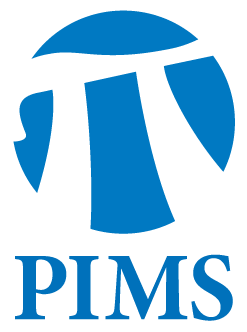## Algebraic Geometry Seminar: Angelo Vistoli

• Date: 03/22/2012
• Time: 15:30
Lecturer(s):
Angelo Vistoli
Location:

University of British Columbia

Topic:

The Nori correspondence

Description:

Abstract:

Let X be a variety over a field k, with a fixed rational point x_0 in X(k). Nori defined a profinite group scheme N(X,x_0), usually called Nori's fundamental group, with the property that homomorphisms N(X,x_0) to a fixed finite group scheme G correspond to G-torsors P --> X, with a fixed rational point in the inverse image of x_0 in P. If k is algebraically closed this coincides with Grothendieck's fundamental group, but is in general very different. Nori's main theorem is that if X is complete, the category of finite-dimensional representations of N(X,x_0) is equivalent to an abelian subcategory of the category of vector bundles on X, the category of essentially finite bundles.After describing Nori's results, I will explain my work in collaboration with Niels Borne, from the University of Lille, in which we extend them by removing the dependence on the base point, substituting Nori's fundamental group with a gerbe (in characteristic 0 this had already been done by Deligne), and give a simpler definition of essentially finite bundle, and a more direct and general proof of the correspondence between representations and essentially finite bundles.

Other Information:

Location: gEOG 147